Select Page

# Line Coding

Line Coding is an encoding technique which is used to convert digital data into digital signal. This process is done at physical layer of OSI Model. Because OSI model is used in digital communication to transmit data from source to destination.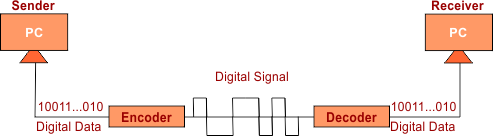## Important Terms

• Data Element: A data element is the smallest piece of information that we want to send i.e. bit 0 or 1.
• Signal Element: It is a shortest element of signal.
• Data Rate: Data elements send in one second. It is measured in bit/sec
• Signal Rate/Baud rate: Signal elements send in one second. SI unit is baud.

Important: Our basic need in encoding to transfer maximum bits (data element) per signal element. So, According to different encoding techniques (Explain below) one or more than one bits can be transferred in one signal element. Sometimes more than one signal elements are required to transmit one bit.

According to Manchester encoding, Following figure explain all above factors.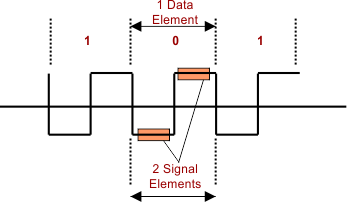## Reasons of Line Coding

To resolve the following factors we use the line coding

### I. Bandwidth Problem

High bandwidth will be required when one digital bit is transferred through more than one element of signal.

• For bit 0 half of the signal is represented by -V and half by zero voltage.
• for bit 1 half of the signal is represented by +V and half by zero voltage
• Example: Data = 01001.

### II. DC Problem

• Due to series of same bit values constant rate is generated. It is called DC component which is difficult to handle. As in the following diagram continuous of 1. So to Eliminate the DC factor Line coding is used.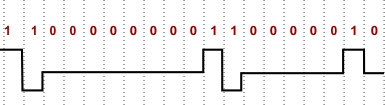### III. Synchronization Problem

When a digital signal represent its beginning, mid and end for each digital bit then there will be no synchronization problem. in the following diagram there is no synchronization problem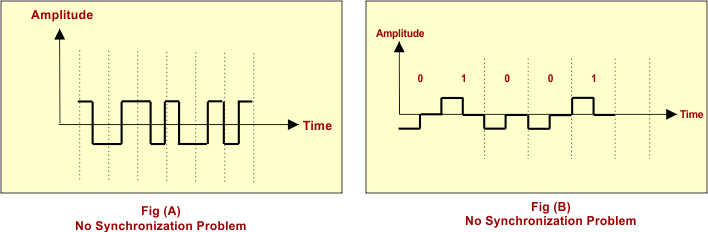But in the following cases there will be the problem of process synchronization because mid points of each signal element is not defined.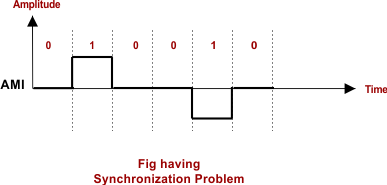Help Other’s By Sharing…